# Backpropagation

Backpropagation has several related meanings when talking about neural networks. Here we mean the process of finding the gradient of a cost function. The rest of this page is somewhat of a tutorial of backpropagation intended for strongly-typed semi-pedantic semi-Vipudlian minds.

## Terminology confusion

• Some people use "backpropagation" to mean the process of finding the gradient of a cost function. This leads people to say things like "backpropagation is just the multivariable chain rule".
• Some people use "backpropagation" or maybe "backpropagation algorithm" to mean the entire gradient descent algorithm, where the gradient is computed using the multivariable chain rule.

Occasionally one hears that backpropagation is "just the multivariable chain rule". However, I think backpropagation is a little trickier than that. Some things that make backpropagation tricky are (these points are probably difficult to appreciate until one has gone through the guts of backpropagation...):

1. You must modify the neural network to get the actual computational graph you need to do the chain rule (i.e. you must modify the neural network graph to add the weights and biases). ("computational graph" refers to a visualization technique used to help with knowing which partials to take in applying the chain rule)
2. The cost isn't a single function, but a sequence of functions (or more like a sequence of compositions) that changes for each layer of the network. So you're not doing the chain rule once and for all; rather, you're doing the chain rule as part of an inductive argument.
3. For each weight, you want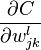$\frac{\partial C}{\partial w^l_{jk}}$ which is like doing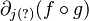$\partial_{j(?)}(f\circ g)$ for some appropriate$f,g$ (for that layer). So it's not the whole chain rule, but rather a single partial derivative of a composition.
4. The cost function is normally seen as a function of the inputs (x's) to the network. But in backpropagation, we leave x fixed and look at C as the w's and b's change. So it takes some extra mental effort to keep this in mind (because the notation usually does not help make this clear).
5. You're not just applying the chain rule. Again, you're doing this as part of an inductive argument, so you must make sure that on the$l$th layer you're only computing the derivatives in terms of later derivatives and values you computed in the forward pass.

## Table of notation

The following table summarizes the notation used on this page. In the course of the page the notation will slowly be introduced, so this table isn't strictly necessary, but it is a handy reference.

Name Symbols Type Explanation
The input to the neural network$x$ Vector For example, this could be the pixel values of a grayscale MNIST image.
The actual output of the neural network (input is implicit)$y$ Vector For example, this could be a vector of length ten, where each coordinate$y_j$ is the probability that the given MNIST digit is$j$.
Number of layers in the neural network$L$ Positive integer For example, if the neural network has one input layer, one hidden layer, and one output layer, then we have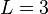$L=3$.
A specific layer of the neural network$\ell$ Positive integer While$L$ is constant for the network, we think of$\ell$ as some specific layer of the network, for example when we are interating over layers.
The weights of the neural network$W$ Vector
The biases of the neural network$b$ Vector
The number of weights in the network, across all layers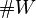$\#W$ Positive integer
The number of biases in the network, across all layers$\#b$ Positive integer
The weights of the neural network on layer$\ell$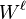$W^\ell$ Matrix (or vector) It is notationally convenient for$W^\ell$ to be a matrix, but you might notice that$W$, the collection of all weights, is just a list, and$W^\ell$ is only the weights of layer$\ell$, so should be a sublist.
The weight that takes the$k$th neuron in layer$\ell - 1$ to the$j$th neuron in layer$\ell$$w^\ell_{j\leftarrow k}$ Real number The$j$ and$k$ seem reversed because this makes more sense in terms of matrix multiplication. The arrow doesn't need to be there and has no deep meaning; it's just a reminder of which neuron goes to which.

## The cost function

The cost function is defined as: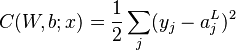$C(W,b;x) = \frac{1}{2} \sum_j (y_j - a^L_j)^2$

Here$y_j$ are the components of the actual value given input$x$, and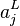$a^L_j$ are the activations in the final (output) layer.

What is the type of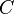$C$? In other words, what are the domain and range of$C$? You might think this is a weird question. Why am I asking the reader to tell me the type of some object? Shouldn't the writer specify the type in the course of defining the object? But actually this sort of thing seems common, where the writer just defines things loosely and leaves the reader to infer the types. But since we are strongly typed minds, let's explicitly think through the type of$C$.

The cost should be a real number, so the range is$\mathbf R$, the set of real numbers. As for the domain, we want to take in all the weights and biases of the network. In each layer, the weights can be thought of as a matrix$W^\ell$, which has some number of rows and columns. The rows and columns of the weight matrices don't have to be the same across layers (except to make the dimensions check out when multiplying and adding adjacent layers). But what dimension does a list of matrices, each with potentially different dimensions, have? We could somehow take the largest row count and column count, then multiply those together and then by$L$. But then there would be a lot of "blanks" where the dimensions are smaller than this maximum. This approach actually seems very clumsy. The right way to think about the domain is to notice that while the weights tend to be packaged together into matrices for each layer, we can easily "unroll" the weights into a single list. Then we can just count the number of weights (which are just real numbers) in all the layers. If that number is$\#W$, we have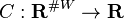$C : \mathbf R^{\#W} \to \mathbf R$. But actually we want to include the biases as well, so if there are$\#b$ biases, we have$C : \mathbf R^{\#W + \#b} \to \mathbf R$.

You might be used to thinking of the cost as a function of the input, i.e.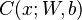$C(x;W,b)$. This turns out to be okay in many situations, but in backpropagation, we want to be differentiating with respect to the weights and biases, while the input remains fixed.

## The point of backpropagation

The whole point of backpropagation is to find the partial derivatives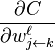$\frac{\partial C}{\partial w^\ell_{j\leftarrow k}}$ which tell us how to adjust the weights of the network. We want to adjust the weights of the network so that the output of the network tends to match the given output. It's easy to get lost in the mess of indices and graphs and nodes and whatnot, and to lose sight of what backpropagation is even supposed to do. Just remember that we have some cost function, some weights, and we want to compute some partial derivatives to plug into gradient descent.

## The usual neural network graph

Neural networks are usually illustrated where each node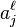$a^\ell_j$ is the activation of neuron$j$ in layer$\ell$. Then the weights are labeled along the edges.

https://gist.github.com/riceissa/95e6171f4bae5ceca034c3cbb9a86508 (file uploads disabled on this wiki so I'll have to host the generated image somewhere)

## Computational graphs

The multivariable chain rule can be represented as a computational graph where the nodes are variables that store values from intermediate computations. Each node can use values given to by edges going into it, and sends its output to nodes going out.

## A different neural network graph

Naively, we might try to piece together the information in the previous sections as follows:

1. The usual neural network graph looks like a computational graph: it's got nodes storing intermediate values that feed into later layers.
2. We know computational graphs are good at representing the process of computing the gradient.
3. In order to do gradient descent, we want the gradient of the cost function.
4. Therefore, by (1)-(3), we can use the neural network graph to represent the process of the computing gradient.

The problem with the above argument is that the computational graph is only good at representing the process of computing the gradient when the variables we are differentiating with respect to are nodes on the graph. But in our case, the cost function is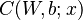$C(W,b;x)$ rather than$C(x;W,b)$, i.e. since we are tinkering with the weights of the network, we want to think of the cost as a function of the weights (parameterized by the input$x$) rather than as a function of the input$x$ (parameterized by the weights$W$ and the biases$b$).

But the usual neural network graph only has nodes for the activations$a^\ell_j$, so how can we compute the relevant derivatives? One solution is to perform surgery on the existing graph to add the weights as nodes.

Now that the weights are on individual nodes, we can think of the activation nodes as receiving all the weights and all the previous activations, then doing the multiplication and passing it through the sigmoid function. This is in contrast to before, where the muliplication was "done along the edges" so that the activation nodes received the products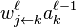$w^\ell_{j\leftarrow k}a^{\ell-1}_k$ and only did the addition and sigmoid.

(But do we even need to put in all the weights? We could mostly ignore all the weights except for the one for which we are computing the partial derivative. For course, if we draw the graph this way, we would have to loop over the weights and modify the graph at each step. Still, the drawing at every step would be simpler.)